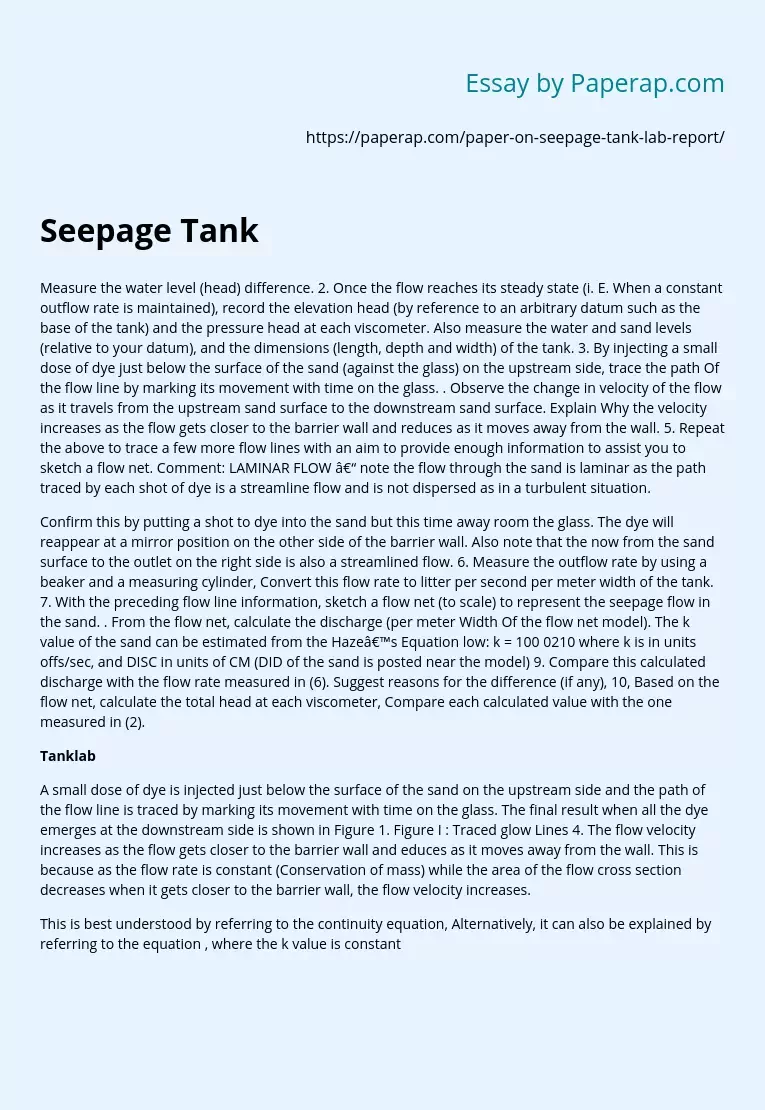# Recording Steady State Water Level Difference

Measure the water level (head) difference. 2. Once the flow reaches its steady state (i. E. When a constant outflow rate is maintained), record the elevation head (by reference to an arbitrary datum such as the base of the tank) and the pressure head at each viscometer. Also measure the water and sand levels (relative to your datum), and the dimensions (length, depth and width) of the tank. 3. By injecting a small dose of dye just below the surface of the sand (against the glass) on the upstream side, trace the path Of the flow line by marking its movement with time on the glass.

. Observe the change in velocity of the flow as it travels from the upstream sand surface to the downstream sand surface. Explain Why the velocity increases as the flow gets closer to the barrier wall and reduces as it moves away from the wall. 5. Repeat the above to trace a few more flow lines with an aim to provide enough information to assist you to sketch a flow net.

Comment: LAMINAR FLOW – note the flow through the sand is laminar as the path traced by each shot of dye is a streamline flow and is not dispersed as in a turbulent situation.

Confirm this by putting a shot to dye into the sand but this time away room the glass. The dye will reappear at a mirror position on the other side of the barrier wall. Also note that the now from the sand surface to the outlet on the right side is also a streamlined flow.

Get quality help nowwriter-CharlotteVerified

Proficient in: Civil Engineering4.7 (348)

“ Amazing as always, gave her a week to finish a big assignment and came through way ahead of time. ”+84 relevant experts are online

6. Measure the outflow rate by using a beaker and a measuring cylinder, Convert this flow rate to litter per second per meter width of the tank. 7. With the preceding flow line information, sketch a flow net (to scale) to represent the seepage flow in the sand. . From the flow net, calculate the discharge (per meter Width Of the flow net model). The k value of the sand can be estimated from the Haze’s Equation low: k = 100 0210 where k is in units offs/sec, and DISC in units of CM (DID of the sand is posted near the model) 9. Compare this calculated discharge with the flow rate measured in (6). Suggest reasons for the difference (if any), 10, Based on the flow net, calculate the total head at each viscometer, Compare each calculated value with the one measured in (2).

## Tanklab

A small dose of dye is injected just below the surface of the sand on the upstream side and the path of the flow line is traced by marking its movement with time on the glass. The final result when all the dye emerges at the downstream side is shown in Figure 1. Figure I : Traced glow Lines 4. The flow velocity increases as the flow gets closer to the barrier wall and educes as it moves away from the wall. This is because as the flow rate is constant (Conservation of mass) while the area of the flow cross section decreases when it gets closer to the barrier wall, the flow velocity increases.

This is best understood by referring to the continuity equation, Alternatively, it can also be explained by referring to the equation , where the k value is constant while the hydraulic gradient i defined by – AL/L increases because the head difference is constant while the flow length L becomes shorter when it gets closer to the barrier wall. 5. The final result when all the dye emerges at the downstream side is shown in Figure I _ 6.

The outflow rate is measured by using a measuring cylinder _ It is measured 3 times and averaged for a more accurate result. The results are shown in Table 2. Measurement Average Flow rate (l,’sec) 4. 17 Table 2 : Flow rate measurement The result is converted to mm/sec per unit width as shown below 7. With the preceding flow net information, a flow net is sketched and presented in Figure 3 of the attached Appendix 1. 8. From the flow net in Figure 3, the flow rate is calculated as shown below.